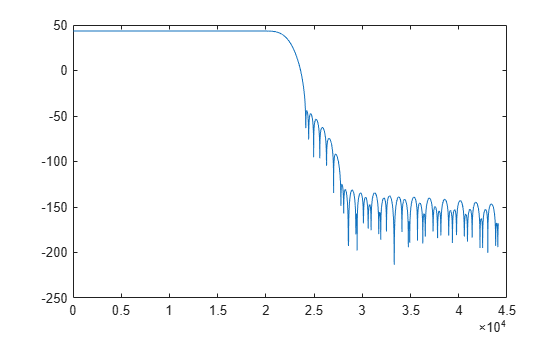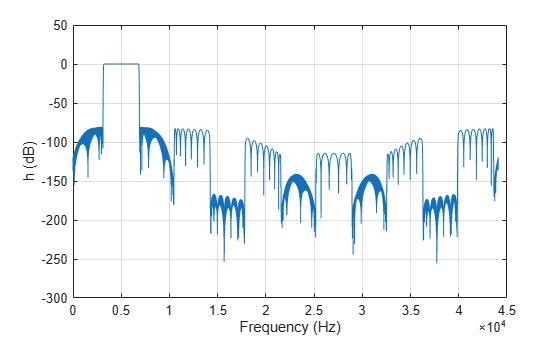# freqz

Frequency response of the multirate multistage filter

## Syntax

``[h,f] = freqz(sysobj,n,range)``
``[h,f] = freqz(sysobj,f)``

## Description

example

````[h,f] = freqz(sysobj,n,range)` returns the complex frequency response, `h`, of the multirate multistage filter System object™ and the frequency vector `f` at which `h` is computed. `n` is the number of frequency points, and `range` is the frequency range over which the response is computed.For the sample rate converter object, the sample rate is the larger of `InputSampleRate` and `OutputSampleRate`.```

example

````[h,f] = freqz(sysobj,f)` returns the complex frequency response `h` computed at the frequency points specified by vector `f`. The input vector `f` is in Hz.```

## Examples

collapse all

Create a multistage sample rate converter with default properties, corresponding to the combined three filter stages used to convert from 192 kHz to 44.1 kHz. Compute and display the frequency response.

```src = dsp.SampleRateConverter; [H,f] = freqz(src); plot(f,20*log10(abs(H)))```Compute and display the frequency response over the range between 20 Hz and 44.1 kHz.

```f = 20:10:44.1e3; [H,f] = freqz(src,f); plot(f,20*log10(abs(H)))```Compute the complex frequency response of a complex bandpass decimator using the `freqz` function.

Create a `dsp.ComplexBandpassDecimator` object. Set the `DecimationFactor` to 12, the `CenterFrequency` to 5000 Hz, and the `SampleRate` to 44100 Hz. Compute and display the frequency response.

```cbp = dsp.ComplexBandpassDecimator(12,5000,44100); [h,f] = freqz(cbp); plot(f,20*log10(abs(h))) grid on xlabel('Frequency (Hz)') ylabel('h (dB)')```## Input Arguments

collapse all

Filter System object, specified as a `dsp.ComplexBandpassDecimator` or a `dsp.SampleRateConverter` System object.

Number of frequency points for response evaluation, specified as a positive integer scalar. If `n` is not specified, the default is 8192.

Data Types: `single` | `double`

Range considered when computing the frequency response, specified as either `'half'` (from 0 to π) or `'whole'` (from 0 to 2π). If `range` is not specified, the defaults is `'half'`.

Frequencies in Hz at which the response is computed, specified as a vector.

Data Types: `double`

## Output Arguments

collapse all

Complex frequency response, returned as a vector.

Data Types: `double`
Complex Number Support: Yes

Frequencies at which the response is computed, returned as a vector.

Data Types: `double`

Introduced in R2018a

## SupportGet trial now